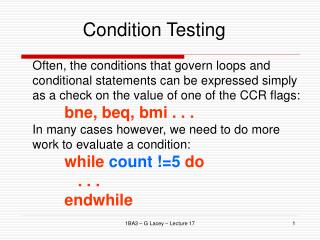# Condition Testing - PowerPoint PPT PresentationDownload PresentationCondition Testing

Condition Testing
Download Presentation## Condition Testing

- - - - - - - - - - - - - - - - - - - - - - - - - - - E N D - - - - - - - - - - - - - - - - - - - - - - - - - - -
##### Presentation Transcript

1. Condition Testing Often, the conditions that govern loops and conditional statements can be expressed simply as a check on the value of one of the CCR flags: bne, beq, bmi . . . In many cases however, we need to do more work to evaluate a condition: while count !=5 do . . . endwhile 1BA3 – G Lacey – Lecture 17

2. CMP In this case, we must subtract 5 from count and then check the Z flag (note: we don’t want to alter count): while move.w #\$05,d1 sub.w d0,d1 * while count!=5 beq endwhile ... endwhile ... As this operation is so common in 68000 programming, an instruction is provided which does exactly that; the cmp instruction. 1BA3 – G Lacey – Lecture 17

3. CMP CMP subtracts the source from the destination, sets CCR accordingly but does not store the result. while cmp.w #5,d0 * while count!=5 beq endwhile ... endwhile ... The CCR flags are set as if the instruction were: sub.w \$5,d0 1BA3 – G Lacey – Lecture 17

4. Greater than and Less than comparison Greater than and less than comparison can be extracted from a combination of CCR flags: 1BA3 – G Lacey – Lecture 17

5. bhi and bge Examples: cmp.b #5,d0 * Unsigned bhi higher -> branch if d0 is higher than 5 cmp.b d0,d1 * Signed bge greater or equal -> branch if d1  d0 1BA3 – G Lacey – Lecture 17

6. Example (bhi) Unsigned: bhi will branch if C = 0 and the Z = 0 i.e. !C & !Z move.w #\$10,d0 cmp.b #\$11,d0 bhi higher ... higher d0 -> 0000 1010 test value -> 0000 1011- 1111 1111 N = 1; B = 1 -> C =1; Z = 0 !C & !Z = !1 & !0 = 0 & 1 = 0 -> is not higher -> branch is not taken 1BA3 – G Lacey – Lecture 17

7. Example (bls) Unsigned: bls will branch if C = 1 or Z = 1 i.e. C | Z move.w #\$10,d0 cmp.b #\$11,d0 bls lower_s ... lower_s d0 -> 0000 1010 test value -> 0000 1011- 1111 1111 N = 1; B = 1 -> C =1; Z = 0 C | Z = 1 | 0 = 1 -> is lower or same -> branch is taken 1BA3 – G Lacey – Lecture 17

8. Example (if): • Slightly more complicated IF condition • If the byte in \$2000 is a valid BCD then store 1 in \$2001 otherwise store a 0. • BCD -> Values of each nibble < 10 • Binary - Coded Decimal (BCD) 1BA3 – G Lacey – Lecture 17

9. Solution in English • Isolate the lower 4 bits. • Isolate the upper 4 bits. • If both values < 10 • Then the result is 1 • Otherwise the result is 0 1BA3 – G Lacey – Lecture 17

10. Pseudo-Code lower = (\$2000); upper = (\$2000); lower = lower & %00001111 upper = upper & %11110000 upper = upper >> 4; if (upper < 10 AND lower < 10) result = 1; else result = 0; endif; (\$2001) = result; We can have arbitrary complexity in the condition. 1BA3 – G Lacey – Lecture 17

11. Pseudo-Code converted to Assembly move.b \$2000,d0 * get lower move.b d0,d1 * get upper and.b #\$0f,d0 * lower 4 bits and.b #\$f0,d1 * upper 4 bits lsr.b #4,d1 cmp.b #9,d0 * if (lower<10 bhi ELSE cmp.b #9,d1 * and upper<10) bhi ELSE move.b #1,d2 * result = 1 bra ENDIF * else ELSE move.b #0,d2 * result = 0 ENDIF move.b d2,\$2001 * store result 1BA3 – G Lacey – Lecture 17

12. Test Condition, Decrement and Branch a = 10; b = 0; do { b = b + c; a = a -1; } while (a >= 0); result = b; This is a very common construct in programming. The MC68332 provides an instruction which implements the decrement and the condition in one: DBcc 1BA3 – G Lacey – Lecture 17

13. Example move.w #10,d0 * a = 10; move.w #0,d1 * b = 0; doloop * do { add.w d2,d1 * b = b + c; dbra d0, doloop * } while (--a>=0) move.w d2,\$2000 * result = b; 1BA3 – G Lacey – Lecture 17

14. Note: • The dbcc command allows the use of 2 loop guards at the same time: • The Condition • The Counter Value • In the above example a dbra was used, as the loop was guarded be the count register d0 only 1BA3 – G Lacey – Lecture 17

15. Example An example of both conditions (the counter and the condition test) being used simultaneously is the following: move.w #10,d0 * a = 10; move.w #0,d1 * b = 0; DOLOOP * do { add.w d2,d1 * b = b + c; dbvs d0, DOLOOP * } while (--Cnt>=0 * AND !Overflow) move.w d2,\$2000 * result = b; The dbvs above may be read as “decrement and branch until overflow set or register value less than 0”. 1BA3 – G Lacey – Lecture 17